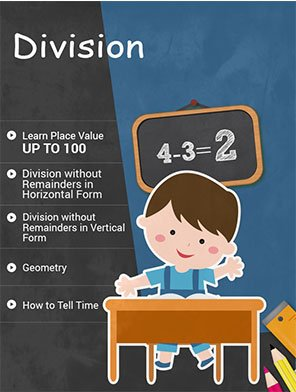\$7.00

## MATH EQUATIONS WORKSHEETS

An open statement in which two expressions are equal is called an equation. Students start this section finding values in the equations. They evaluate algebraic expressions and simplify them to be preparing to solve the equations. After the discussion of properties of equations, students practice solving simple equations, equation with parentheses, variables on two sides of the equations, and word problems of equations related to time and percent.

Worksheet # Topics/Curriculum # of Pages 2pg Sample Add to cart
Unit-1 Finding Values in Equations 8 pages\$1.00

Unit-2 Evaluating Algebraic Expressions 8 pages\$1.00

Unit-3 Adding and Subtracting Algebraic Expressions 8 pages\$1.00

Unit-4 More Problems on Adding and Subtracting Algebraic Expressions 8 pages\$1.00

Unit-5 Simplifying Algebraic Expressions 8 pages\$1.00

Unit-6 Solving Equations 8 pages\$1.00

Unit-7 More Problems on Solving Equations 8 pages\$1.00

Unit-8 Solving Equations with Parentheses 8 pages\$1.00

Unit-9 Advanced Problems on Solving Equations 8 pages\$1.00

Unit-10 Word Problems on Equations 8 pages\$1.00